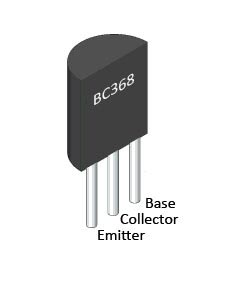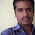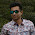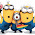## Clap Switch Circuit Diagram Project

This is a simple clap switch circuit diagram project. If you are a beginner electronics learner, and love to do new project experiment then this is a great circuit for you.  This circuit can on/off a 220V electronic device like fan, lamp by the sound of clap.

### Watch The Video Of Clap Switch Circuit Diagram Project

#### Circuit Description

As shown in the circuit a very few number of parts is used including relay, transformer, condenser microphone, few transistor, a lamp etc. Lamp is used as a testing device; here another device could be use like fan, calling bell, radio etc.

The basic principle applied in this circuit:
“Clap sound is being converted into electrical signal and a relay gets triggered from it and controls the load.”

A small microphone (MIC) detects the clap sound and converts into electrical signal. Which is amplified by Q1 (here Q1 used as a common emitter amplifier). Amplified signal goes into the Bistable Multivibrator section made by two transistors Q2 and Q3.

When we apply a trigger to the Bistable Multivibrator circuit with a clap sound then, if Q2 is on, Q3 gets switched off. Thus, the circuit remains stable in a single state continuously. At the moment if we trigger with another clap sound the two states will flip-flop with Q2 switched off and Q3 switched on. The circuit will remain stable continuously until we apply the next trigger.

Relay gets triggered from the output of current-amplifier (Q4) which is used to amplify the flip-flop signal. Relay is kind of electromagnetic switch, which is used to controlling other high voltage (AC) electrical appliance (Load in our circuit) with this low voltage (DC) clap switch.

### Part List:

R1 = 15KΩ Resistor
R2, R11, R12 = 2.2MΩ Resistor
R3 = 270KΩ, R4 = 3.3KΩ Resistor
R5, R6 = 1.5KΩ Resistor
R13 = 2.2KΩ Resistor
R3 = 270KΩ Resistor
R4 = 3.3KΩ Resistor
R7, R8 = 10KΩ Resistor
R9, R10 = 27KΩ Resistor

C1 = 1000µf/16v Capacitor
C2 = .01µf Capacitor
C3, C4 = .047µf Capacitor

Q1, Q2, Q3 = BC548 Transistor
Q4 = BC368 Transistor
D2, D3, D4 = IN 4148 Diode
D1, D5 = IN 4007 Diode

MIC = Condenser Microphone
RLY = 12V Relay
Load = AC 220V Bulb or Fan
Tx = 12v/0.5A Transformer

#### BC548 and BC368 Transistor PinoutReference: Bistable Multivibrator [wikipedia]

1.1.2.